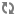Tuning Topologies# Tune Parser Kafka Partitions

When you tune a new parser, the first variable that you should determine is the minimum number of Kafka partitions required.

1. Create a Kafka topic with a single partition.
2. Run the Kafka producer for a set amount of time.
For example, 10 minutes.
3. Calculate the approximate number of events per second based on the total size of the Kafka partition.
4. Launch the parser toplogy with the following:
• 1 spout
• 1 worker
• Several parser executers (10 or more)
5. Let the parser run for a set amount of time.
6. If the parser executors reach capacity, increase the number of executors and restart.
When you restart the toplogies, ensure that the Kafka offset strategy is set to "LATEST".
7. Calculate the approximate number of events per second from the statistics in the Storm user interface.
8. If the events in the Kafka topic are fully processed by the parser topology before the set amount of time is complete, you can omit the events per second calculation and instead use the first result.
For example:
``Num partitions = t/p``
The number of partitions should be proportional to the number of Storm nodes. Because Kafka partitions are tied to the number of Kafka spouts, which need to be evenly distributed between Storm workers, the number of partitions should be divisible by the number of Storm workers.